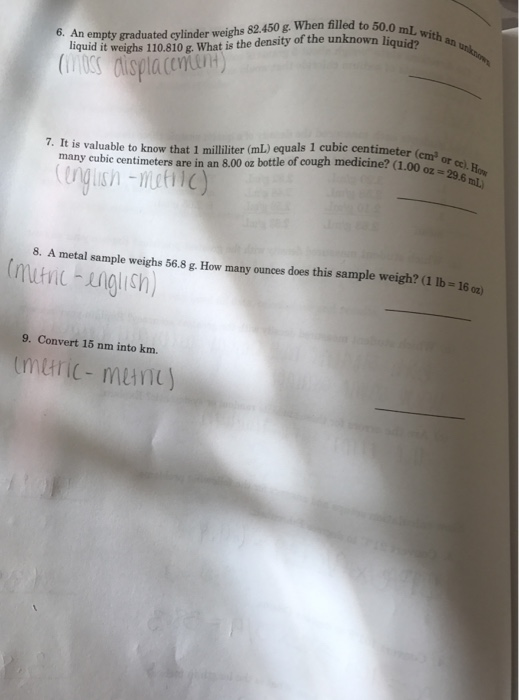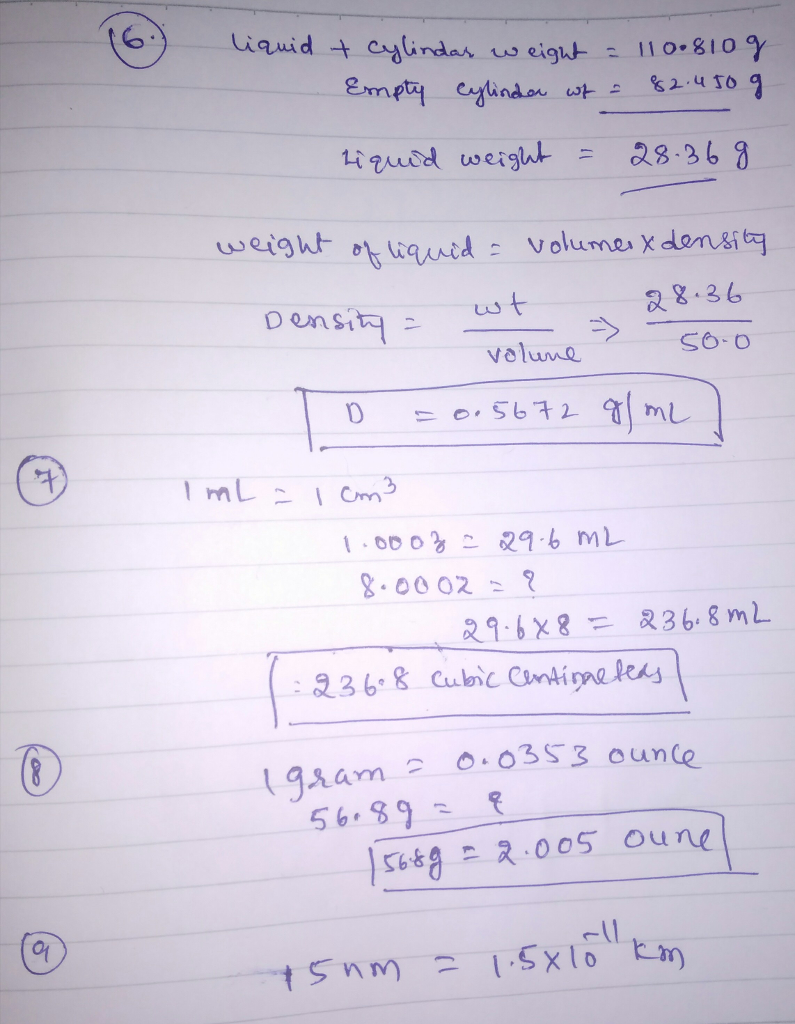# Homework Solution: An empty graduated cylinder weighs 82.450 g. When filled to 50.0 mL with an…An empty graduated cylinder weighs 82.450 g. When filled to 50.0 mL with an unknown liquid it weighs 110.810 g. What is the density of the unknown liquid? It is valuable to know that 1 milliliter (mL) equals 1 cubic centimeter (cm^3 or cc). How many cubic centimeters are in an 8.00 oz bottle of cough medicine? (1.00 oz = 29.6 mL) A metal sample weighs 56.8 g. How many ounces does this sample weigh? (1 lb = 16 oz) Convert 15 nm into km.